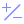#Log (PQL)

Returns the natural logarithm (base e) of a number.

• Returned Output: Numeric
• Library: PQL \ Common \ Math
• Version: 2018.00.000
• Compatibility:
• Can be combined with and other PQL function throughout the application.
• It CANNOT be used with MDX or VBA functions. But it can be used on MDX-based content in other parts of the application.

#### Syntax

Log( <Numeric> )

* Click on the function's arguments above for more details on the input values.

• The numeric value cane be any type of positive number: integer, float etc.
##### Different Function types
• This function is like the Excel LN function.
• Also see the exponent and e functions.

#### Examples

This example returns "1"

Log(e())

This example returns "4.814"

Log(123.3)